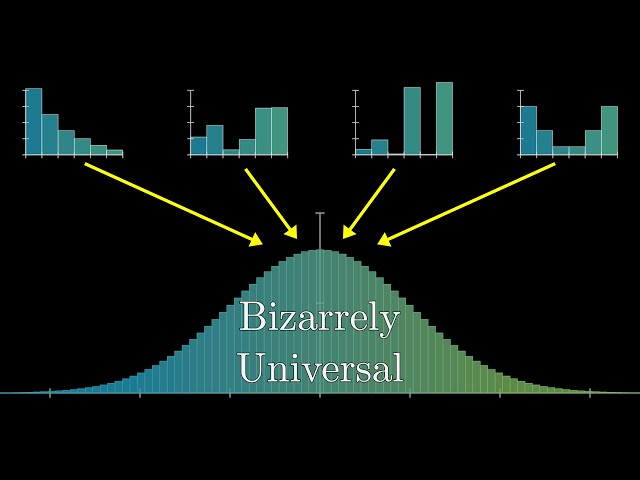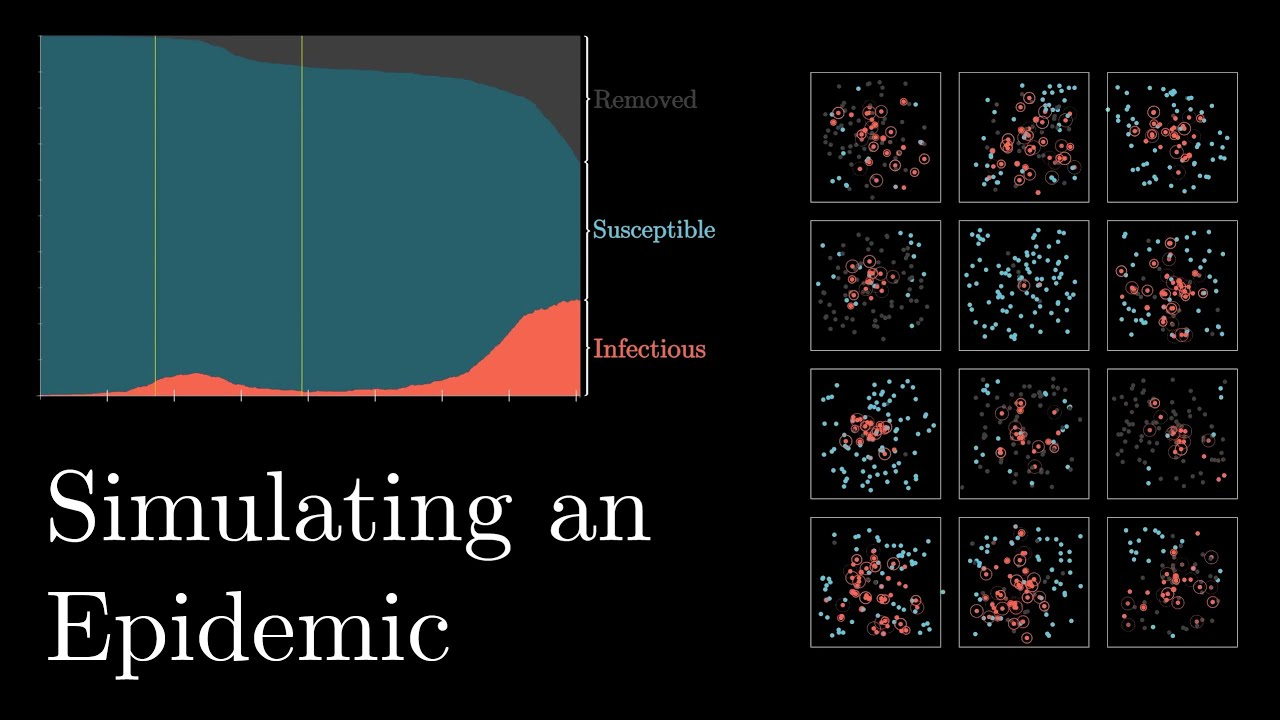# 3 brown 1 blueMathematics Statistics

#### But what is the Central Limit Theorem?

3Blue1Brown provides a visual introduction to probability’s most important theorem.Mathematics

#### The mathematically optimal Wordle strategy

An excuse to teach a lesson on information theory and entropy.Mathematics

#### Why Do Prime Numbers Make These Spirals?

3Blue1Brown  explores a fascinating property of prime numbers.Mathematics Science

#### Simulating an Epidemic

3Blue1Brown walks through the mathematics of epidemics through simulations. The source code for this video is visible here: https://github.com/3b1b/manim/blob/shaders/from_3b1b/active/sir.py